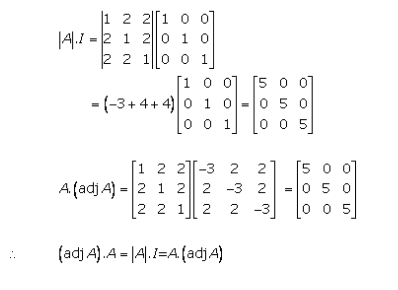# RD Sharma Solutions Class 12 Adjoint And Inverse Of A Matrix Exercise 7.1

## RD Sharma Solutions Class 12 Chapter 7 Exercise 7.1

### Exercise 7.1#### Practise This QuestionMention the proper order of identifying a point D on BC such that Ar(ABD)Ar(ADC)=23.
1) Join B5C and draw a line parallel to B5C from B2.
2) Identify the ratio in which the point D divides BC using the relation given.
3) Mark 5 points B1, B2, B3, B4 and B5 on BX such that they are equidistant.
4) Construct a ray BX which makes an acute angle with line segment BC.
5) The point of intersection of the parallel line from B2 with BC is the point D.